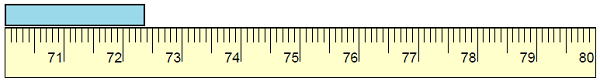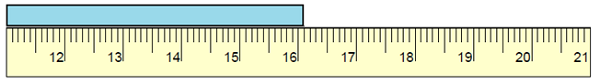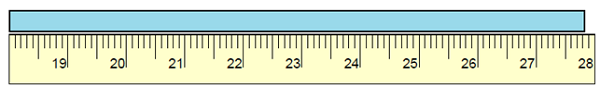# Measuring Length to the Nearest Centimeter

We can measure how long things are, or how tall, or how far apart they are. Those are all examples of length measurements.

Metric rulers are base 10. So, using metric rulers, we can measure lengths to the nearest centimeters and nearest millimeters. Meanwhile in foot/inch rulers we can measures to nearest half inch, quarter inch and so on.

In this lesson, we solve problems where the length of objects is measured to the nearest centimeter. This means that the measurement is accurate up to a centimeter. Usually objects like pens, bottles, tennis rackets, rulers and so on have lengths that can be measured conveniently in centimeters.

Find the length of the following object to the nearest centimeter.### Solution

Step 1:

The reading is closer to 72 cm than to 73 cm.

Step 2:

So, the length of the given object to the nearest centimeter is 72 cm.

Find the length of the following object to the nearest centimeter.### Solution

Step 1:

The reading is closer to 16 cm than to 17 cm.

Step 2:

So, the length of the given object to the nearest centimeter is 16 cm.

Find the length of the following object to the nearest centimeter.### Solution

Step 1:

The reading is closer to 28 cm than to 27 cm.

Step 2:

So, the length of the given object to the nearest centimeter is 28 cm.